# 可视化工具不知道怎么选？深度评测5大Python数据可视化工具！

```【摘要】相信很多读者学习Python就是希望作出各种酷炫的可视化图表，当然你一定会听说过 Matplotlib 、 Pyecharts 、 Seaborn 、 Plotly 、 Bokeh 这五大工具，本文就将通过真实绘图来深度评测这五个Python数据可视化的库， 看看到底这几种工具各有什么优缺点，在制作图表时该如何选择。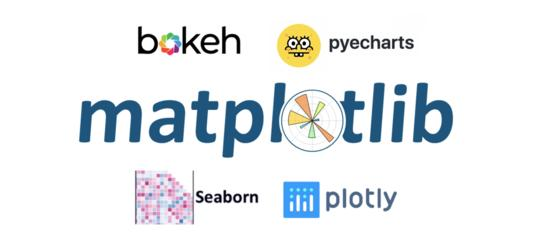# 指标说明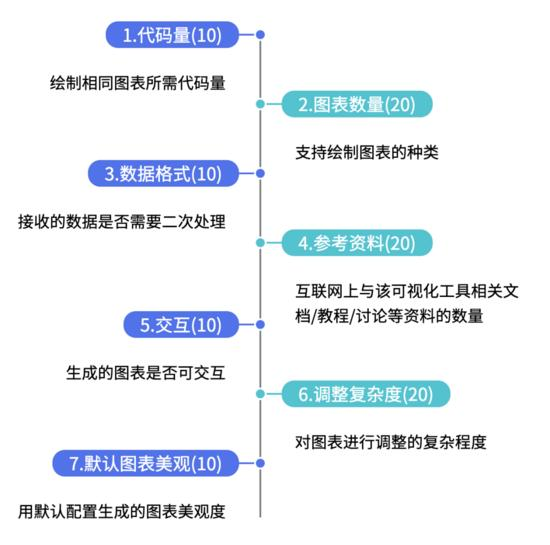# 数据说明

```from pyecharts.faker import Faker
x = Faker.choose()
y1 = Faker.values()
y2 = Faker.values()```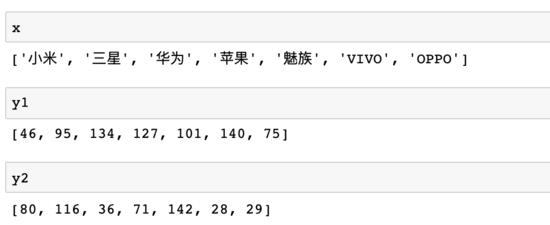x为一列品牌名称，y1/y2为一列相同长度的 无意义 数据，接下来让我们使用不同的库对这组数据进行可视化！

# Pyecharts

Echarts 是一个由百度开源的数据可视化，凭借着良好的交互性，精巧的图表设计，得到了众多开发者的认可。而Python是一门富有表达力的语言，很适合用于数据处理。当数据分析遇上数据可视化时， pyecharts 诞生了，支持 30+ 种图表 。 在pyecharts中制作条形图首先需要导入相关库

```from pyecharts import options as opts
from pyecharts.charts import Bar```

```c = ( Bar() .add_xaxis(x) .add_yaxis("商家A", y1) .add_yaxis("商家B", y2) .set_global_opts(title_opts=opts.TitleOpts(title="Pyecharts—柱状图", subtitle=""))
).render_notebook()```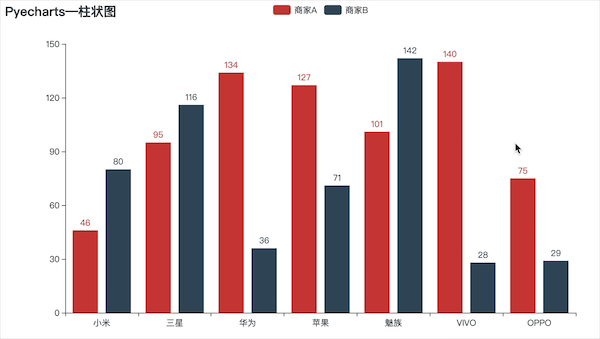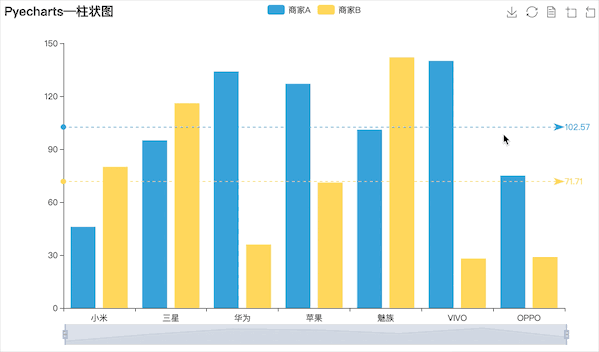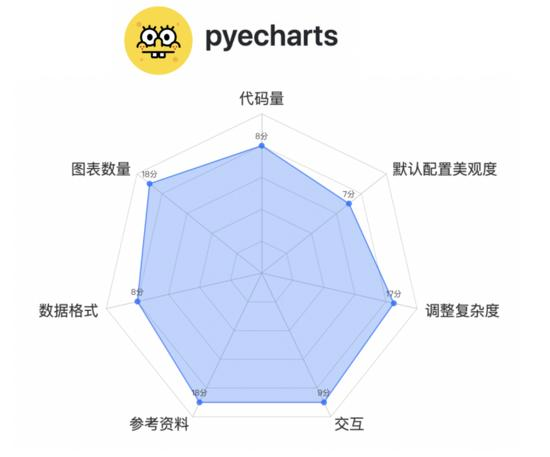# Matplotlib

Matplotlib 应该是最广泛使用的Python可视化工具，支持的图形种类非常多，使用Matplotlib制作相同效果的图需要先导入相关库，并且并不支持原生中文所以还要设置下中文显示

```import matplotlib.pyplot as plt
import numpy as np
plt.rcParams['font.sans-serif'] = ['SimHei'] ```

```width = 0.35
x1 = np.arange(len(x))

fig, ax = plt.subplots()
rects1 = ax.bar(x1 - width/2, y1, width, label='商家A')
rects2 = ax.bar(x1 + width/2, y2, width, label='商家B')

ax.set_title('Matplotlib—柱状图')
ax.set_xticks(x1)
ax.set_xticklabels(x)
ax.legend()

plt.show()```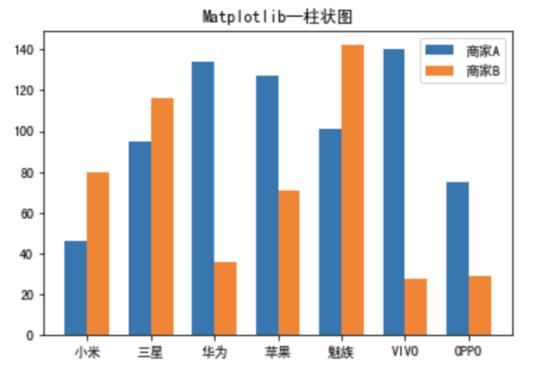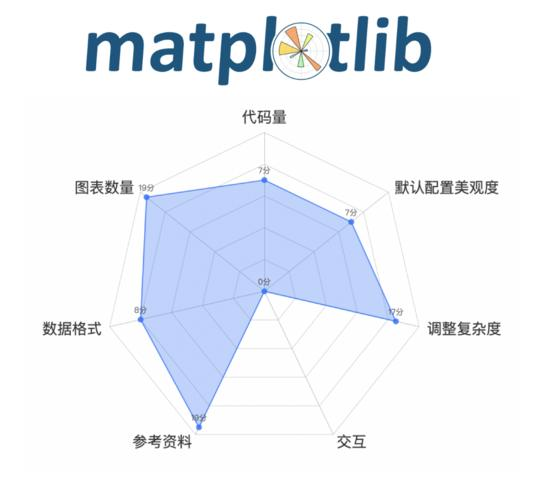# Plotly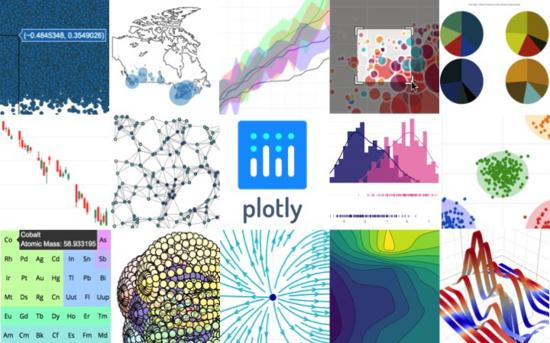Plotly 也是一款非常强大的Python可视化库， Plotly内置完整的交互能力及编辑工具，支持在线和离线模式，提供稳定的API以便与现有应用集成，既可以在web浏览器中展示数据图表，也可以存入本地拷贝 。 但是由于官方 未提供中文文档 ，网上关于Plotly的教程也仅限于官方的一些demo，对于一些详细的参数设置并 没有太多资料 ，首先还是先导入相关库并设置notebook显示

```import plotly
import plotly.offline as py
import plotly.graph_objs as go
plotly.offline.init_notebook_mode(connected=True)```

```trace0 = go.Bar( x = x, y = y1, name = '商家A',
)
trace1 = go.Bar( x = x, y = y2, name = '商家B',
)
data = [trace0,trace1]
layout = go.Layout( title={ 'text': "Plotly-柱状图", 'y':0.9, 'x':0.5, 'xanchor': 'center', 'yanchor': 'top'})
fig = go.Figure(data=data, layout=layout)
py.iplot(fig)```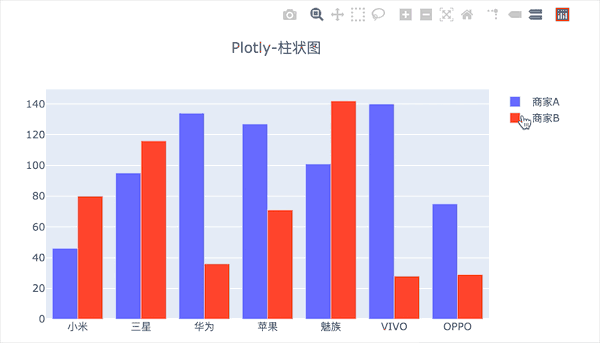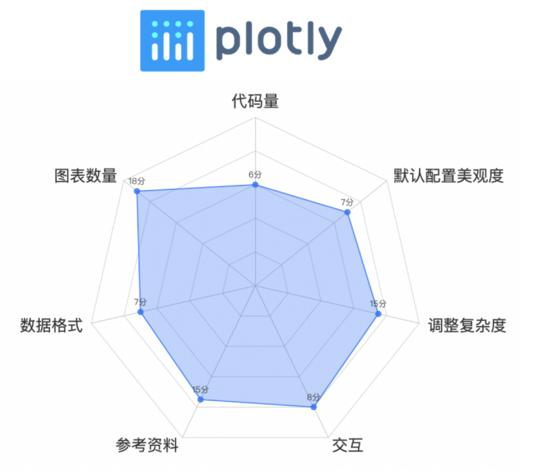# Bokeh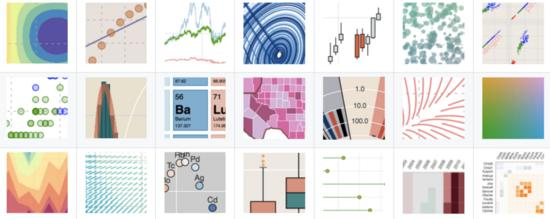Bokeh 是一个专门针对Web浏览器的呈现功能的 交互式 可视化Python库。这是Bokeh与其它可视化库最核心的区别，它可以做出像 D3.js 简洁 漂亮的交互可视化效果，但是使用难度低于D3.js，首先还是导入相关库

```from bokeh.transform import dodge
import pandas as pd
from bokeh.core.properties import value
import numpy as np
import matplotlib.pyplot as plt
%matplotlib inline
from bokeh.io import output_notebook
output_notebook() # 导入notebook绘图模块
from bokeh.plotting import figure,show
from bokeh.models import ColumnDataSource# 导入图表绘制、图标展示模块 # 导入ColumnDataSource模块 # 导入dodge、value模块```

```df = pd.DataFrame({'商家A':y1,'商家B':y2}, index = x_)
_x = ['商家A','商家B'] # 系列名
data = {'index':x_}
for i in _x: data[i] = df[i].tolist()# 生成数据，数据格式为dict
source = ColumnDataSource(data=data)# 将数据转化为ColumnDataSource对象

p = figure(x_range=x_, y_range=(0, 150), plot_height=350, title="boken-柱状图",tools="crosshair,pan,wheel_zoom,box_zoom,reset,box_select,lasso_select")

p.vbar(x=dodge('index', -0.1, range=p.x_range), top='商家A', width=0.2, source=source,color="#718dbf", legend=value("商家A"))
p.vbar(x=dodge('index',  0.1, range=p.x_range), top='商家B', width=0.2, source=source,color="#e84d60", legend=value("商家B"))# dodge(field_name, value, range=None) → 转换成一个可分组的对象，value为元素的位置（配合width设置）
p.xgrid.grid_line_color = None
p.legend.location = "top_left"
p.legend.orientation = "horizontal" # 其他参数设置
show(p)```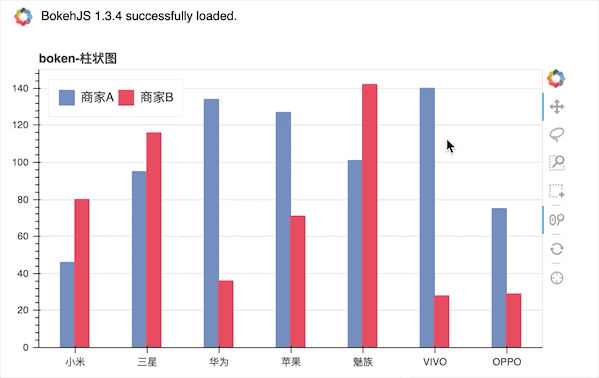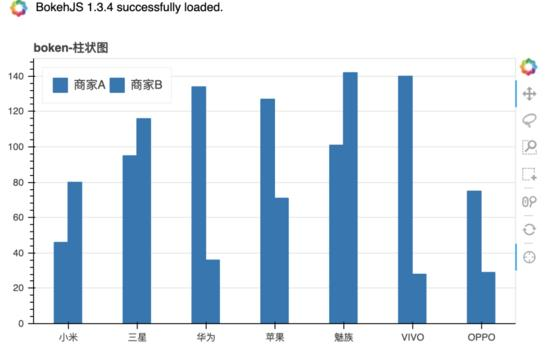Bokeh一个很明显的特点就是 代码量较上面三个工具要多了很多 ，大多是在 数据的处理 上，并且和Plotly一样，有关bokeh相关的 中文资料也不多 ，大多是入门型的基本使用于介绍，虽然从官方给出的图来看能作出很多比pyecharts更精美的图，但是查找相关参数的设置上将会耗费一定时间，主观评分 71 分。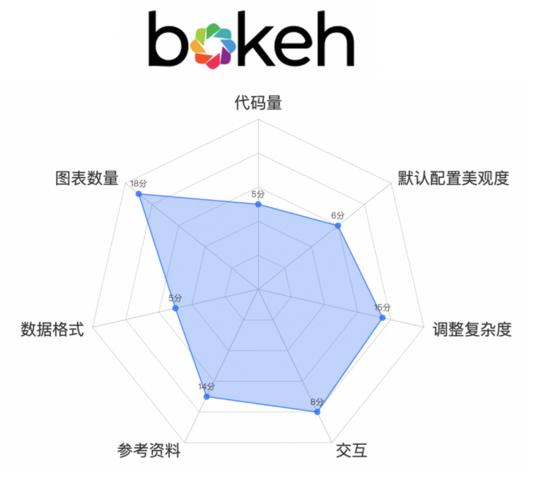# Seaborn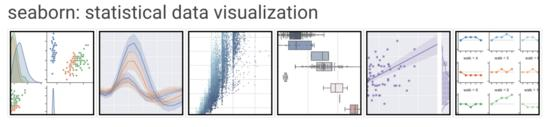```import seaborn as sns
import matplotlib.pyplot as plt
plt.rcParams['font.sans-serif'] = ['SimHei'] ```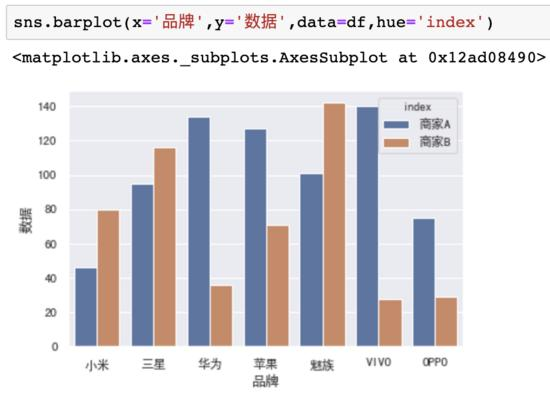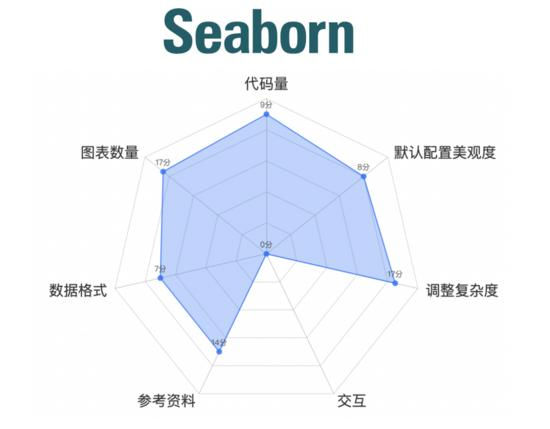# 小结

• Python爬虫urllib模块

Python爬虫练习(urllib模块) 关注公众号“轻松学编程”了解更多。 1、获取百度首页数据 流程：a.设置请求地址 b.设置请求时间 c.获取响应(对响应进行解码) ''' 获取百度首页 ''' imp...

• 【python学习笔记】字典

1.字典的含义 ① 字典是python中唯一的映射类型 ② python的字典为键值对，有些地方称为hash，有些地方称之为关系数组 ③ 映射类型区别于序列类型，序列类型以数组的方式进行存储，通过索引的方式获取相对应位置的值，一般索引与对应位置存储的数据毫无关系 2.创建和访问字典 ① 空字典 >>> empty = {} >>> type(empt...

• 数据安全性强制存取控制

强制存取控制(   Mandatory Access Control    MAC )是指系统为保证更高程度的安全性，按照TDI/TCSEC标准中安全策略的要求所采取的强制存取检查手段。它不是用户直接感知或进行控制的。强制存取控制适用于那些对数据有严格而固定密级分类的部门，例如军事部门或政府部门。 　　 　在强制存取控制中，数据库管理系统所管...

• 大数据学习之路（三）——hadoop分布式

睡不着觉，拉着狗陪我写博客 开始搭建分布式hdfs环境 参考文章 http://hadoop.apache.org/docs/r2.5.2/hadoop-project-dist/hadoop-common/C...

• python的math库函数汇总

目录 一、math库介绍二、math库使用三、math库函数3.1 math库的数字常数（4个）3.2 math库的数值表示函数（16个）3.3 math库的幂对数函数（8个）3.4 math库的三角运算函数（...

• 多测师_Python（while 循环语句）

一.循环语句   1.while 循环 当我们在 python 中需要重复执行一些动作的时候，这时我们就要用到循环while 循环的结构，当条件成立的时候，就会执行里面的代码while 循环不断的运行，直到指定的条件不满足为止。注意死循环 while 条件成立: 执行内容 number ...

• DQL（数据查询语言）下、DML（数据管理语言）)

DQL（数据查询语言）下、DML（数据管理语言）) 多表查询的join on连接 左外连接：left join on 右外连接：right join on 使用on子句的好处： 自然连接中是以具有相同名字的列为连接...

• 深度强化学习DQN（附DQN训练Flappy Bird源代码）

1.DQN算法 关于DQN详细算法请参考： 深度强化学习DQN详解 深度强化学习—DQN 深度强化学习入门 2.DQN源代码 源代码中一共有三个主要.py文件。 BrainDQN_Nature.py和Brain...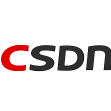### CSDN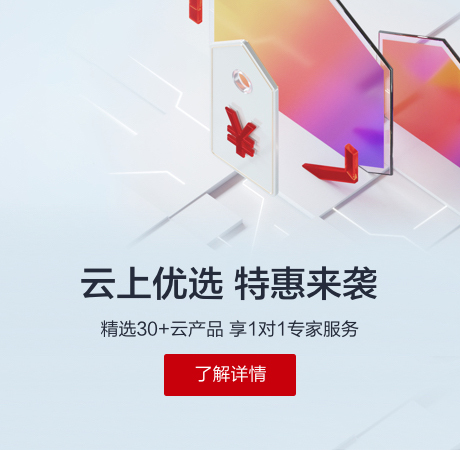5

)